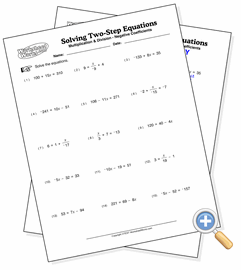# Multiplication & Division

## Solving Two-Step EquationsSolve two-step equations using multiplication and division

These two-step equations are solved by using addition and subtraction, then either multiplication or division to eliminate a denominator or coefficient. All problems resolve to integers. You can adjust the difficulty of the problems by increasing the values size, using negative coefficients and flipping the sides of the equations.